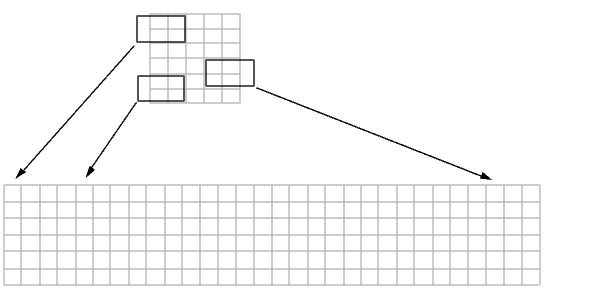Image Processing Toolbox User's GuideSliding Neighborhoods

For a sliding neighborhood operation, `colfilt` creates a temporary matrix that has a separate column for each pixel in the original image. The column corresponding to a given pixel contains the values of that pixel's neighborhood from the original image.

The following figure illustrates this process. In this figure, a 6-by-5 image matrix is processed in 2-by-3 neighborhoods. `colfilt` creates one column for each pixel in the image, so there are a total of 30 columns in the temporary matrix. Each pixel's column contains the value of the pixels in its neighborhood, so there are six rows. `colfilt` zero-pads the input image as necessary. For example, the neighborhood of the upper left pixel in the figure has two zero-valued neighbors, due to zero padding.colfilt Creates a Temporary Matrix for Sliding Neighborhood

The temporary matrix is passed to a function, which must return a single value for each column. (Many MATLAB functions work this way, for example, `mean`, `median`, `std`, `sum`, etc.) The resulting values are then assigned to the appropriate pixels in the output image.

`colfilt` can produce the same results as `nlfilter` with faster execution time; however, it might use more memory. The example below sets each output pixel to the maximum value in the input pixel's neighborhood, producing the same result as the nlfilter example shown in Linear and Nonlinear Filtering.

• ```I2 = colfilt(I,[3 3],'sliding',@max);
```Column Processing Distinct Blocks